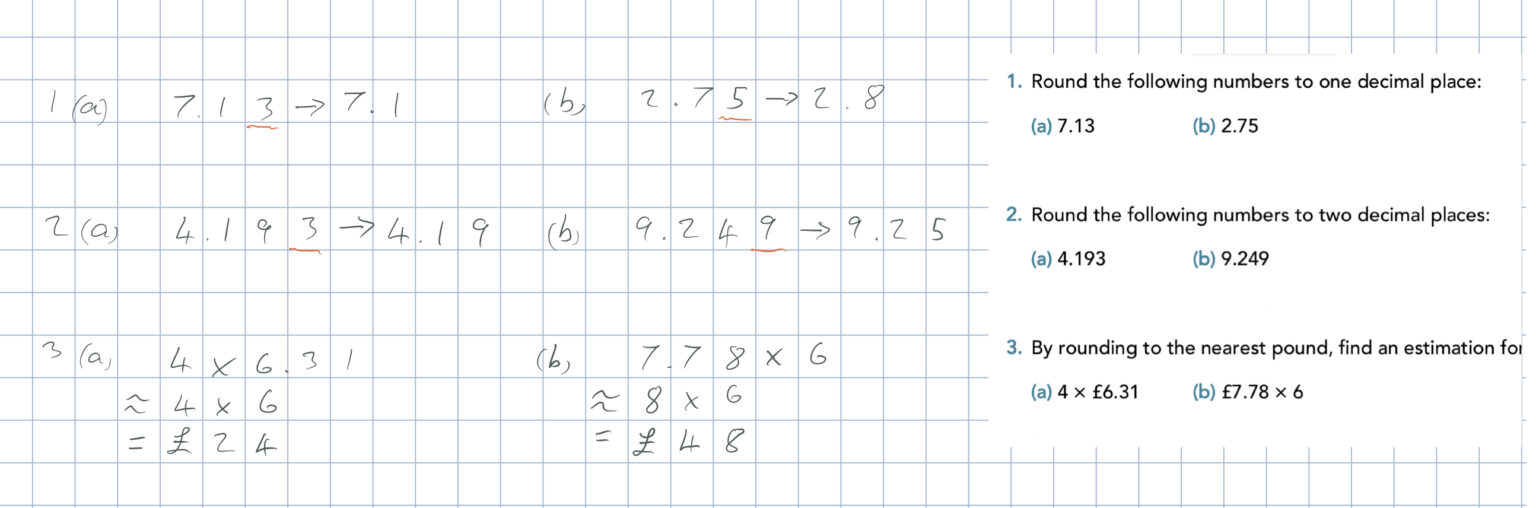When rounding to decimal places, look at the number after the decimal place being rounded. If it is 5 or more, then number is rounded up, if it is less than 5, then number is rounded down (it remains the same).

Rounding for estimation (question 3) is a very useful skill when working with money, as prices can often consist of complicated decimal numbers. So, if we need to estimate how much we are spending at the shops, it is good practice to round to the nearest pound.

When rounding to find an estimate, we can use the ‘is approximately’ sign in line two (see below), then an ‘equals’ sign in line three.